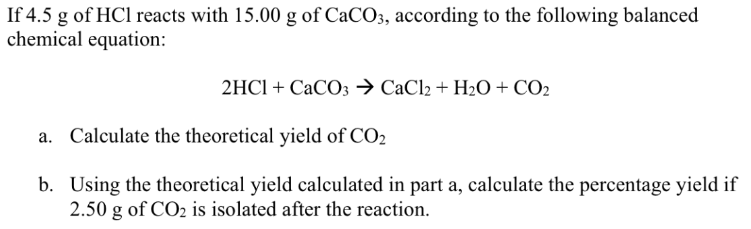# If 4.5 g of HCl reacts with 15.00 g of CaCO3, according to the following balanced chemical equation: 2 HCl + CaCO3 -> CaCl2 + H2O + CO2 a. Calculate the theoretical yield of CO2 b. Using the theoretical yield calculated in part a, calculate the percentage yield if 2.50 g of CO2 is isolated after the reaction.